signed

QiShunwang

“诚信为本、客户至上”

# 张量以及函数

2021/4/26 19:57:31   来源：

### 张量

• 张量模态
• Khatri-Rao积（KR积）
• numpy.trace(a, offset=0, axis1=0, axis2=1, dtype=None, out=None)
• np.linalg.norm(求范数)
• RuntimeWarning: invalid value encountered in double_scalars
• A = np.column_stack((x_vals_column, ones_column))
• flatten()函数用法
• set() 函数
• np.arange函数的使用
• numpy.astype() 转换数据类型
• np.tile
• sum(axis=1)
• Numpy tolist() 用法
• np.c_的用法
• np.argmax
• 查准率(Precision) 、召回率(Recall)与F1分数、准确率(Accuracy) | 查准率(Precision) | 查全率(Recall)
• Recall（召回率） Precision（准确率） F-Measure E值 sensitivity（灵敏性） specificity（特异性）漏诊率 误诊率 ROC AUC
• numpy.around()函数
• python csv.reader() 与 pd.read_csv()的区别
• csv.reader()
• pd.read_csv()
• sp.linalg.solve_sylvester
• numpy.diagflat(a，k = 0)：
• iloc和loc
• pickle.dump
• numpy.nanstd()函数

# 张量模态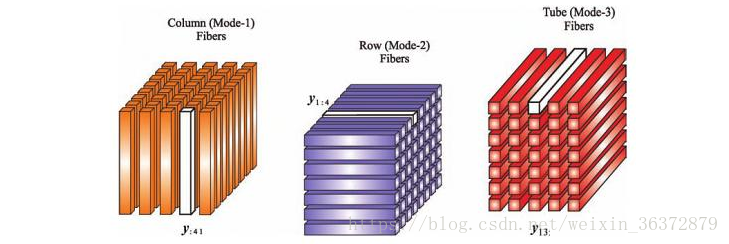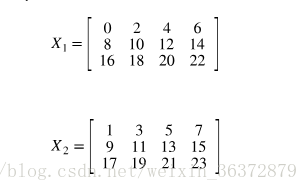``````import numpy as np
import tensorly as tl
X = tl.tensor(np.arange(24).reshape(3, 4, 2))
``````

``````print(tl.unfold(X, mode=0))  #1模展开
print(tl.unfold(X, mode=1))
print(tl.unfold(X, mode=2))
``````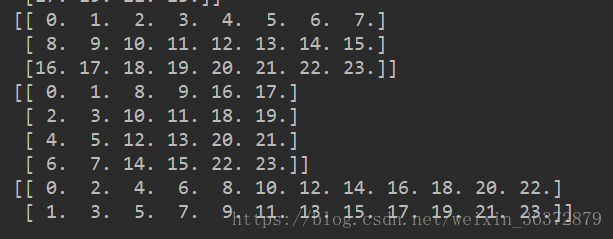``````unfolding = tl.unfold(X, 1)
original_shape = X.shape
tl.fold(unfolding, mode=1, shape=original_sha
``````

# Khatri-Rao积（KR积）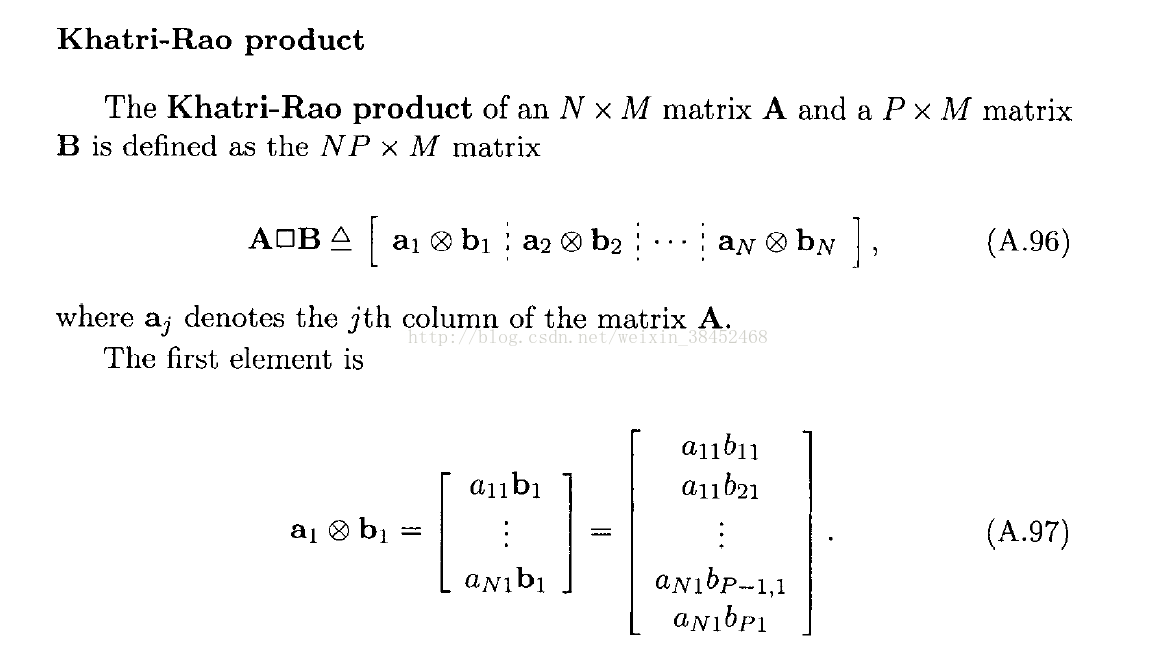A=[1 2;3 4],B=[5 6;7 8]

2的矩阵，得到（2
2）2=42的矩阵。

[15=5
1
7=7
35=15
3
7=21]

[26=12
2
8=16
46=24
4
8=32]

[5 12
7 16
15 24
21 32]
Matlab代码：
for k=1:K %K为输入矩阵的列数
C(:,k)=kron(A(:,k),B(:,k));
end

# np.linalg.norm(求范数)

（1）np.linalg.inv()：矩阵求逆
（2）np.linalg.det()：矩阵求行列式（标量）

`x_norm=np.linalg.norm(x, ord=None, axis=None, keepdims=False)`
①x: 表示矩阵（也可以是一维）
②ord：范数类型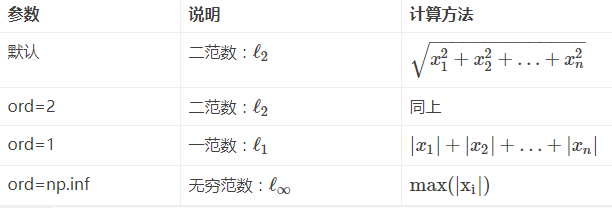ord=1：列和的最大值
ord=2：|λE-ATA|=0，求特征值，然后求最大特征值得算术平方根(matlab在线版，计算ans=ATA，[x,y]=eig(ans)，sqrt(y)，x是特征向量，y是特征值)
ord=∞：行和的最大值
ord=None：默认情况下，是求整体的矩阵元素平方和，再开根号。（没仔细看，以为默认情况下就是矩阵的二范数，修正一下，默认情况下是求整个矩阵元素平方和再开根号）
③axis：处理类型
axis=1表示按行向量处理，求多个行向量的范数
axis=0表示按列向量处理，求多个列向量的范数
axis=None表示矩阵范数。
④keepding：是否保持矩阵的二维特性
True表示保持矩阵的二维特性，False相反

``````x = np.array([
[0, 3, 4],
[1, 6, 4]])
#默认参数ord=None，axis=None，keepdims=False
print "默认参数(矩阵整体元素平方和开根号，不保留矩阵二维特性)：",np.linalg.norm(x)
print "矩阵整体元素平方和开根号，保留矩阵二维特性：",np.linalg.norm(x,keepdims=True)

print "矩阵每个行向量求向量的2范数：",np.linalg.norm(x,axis=1,keepdims=True)
print "矩阵每个列向量求向量的2范数：",np.linalg.norm(x,axis=0,keepdims=True)

print "矩阵1范数：",np.linalg.norm(x,ord=1,keepdims=True)
print "矩阵2范数：",np.linalg.norm(x,ord=2,keepdims=True)
print "矩阵∞范数：",np.linalg.norm(x,ord=np.inf,keepdims=True)

print "矩阵每个行向量求向量的1范数：",np.linalg.norm(x,ord=1,axis=1,keepdims=True)
``````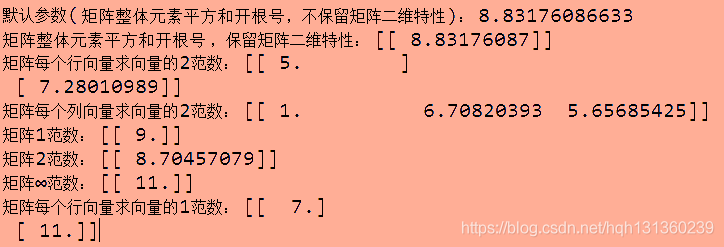# RuntimeWarning: invalid value encountered in double_scalars

• 这是个警告提示(warning)，而不是错误(Error)。所以在代码运行时出现这个提示时，代码仍然可以正常运行。但同时因为他不是错误，所以使用try-except是捕获不了异常的。

• 出现这个提示一般是因为出现了0/0导致的。但是出现分母为0的情况时，没有触发ZeroDivisionError(0除异常)，这一点也很意外。

• 解决方法：使用if-else语句判断当分母为0时重新给id_col赋值。
下面来简单看看ZeroDivisionError异常和RuntimeWarning警告之间的区别，以及在何种情况下会触发这两类提示。

• 当分母0的数据类型为内置数据类型时，可以触发ZeroDivisionError异常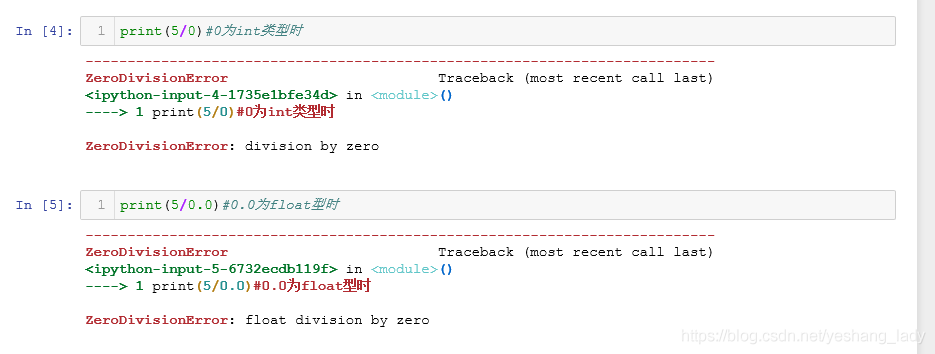• 当分母0为numpy中的数据类型时，触发RuntimeWarning警告。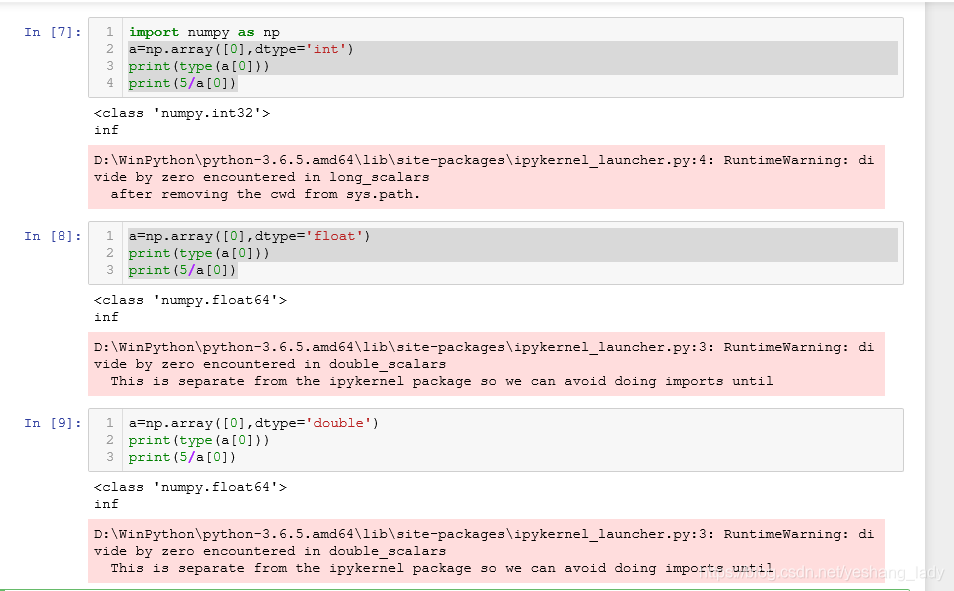• 当内置数据类型和numpy数据类型计算时，其计算结果的类型为numpy中的计算类型。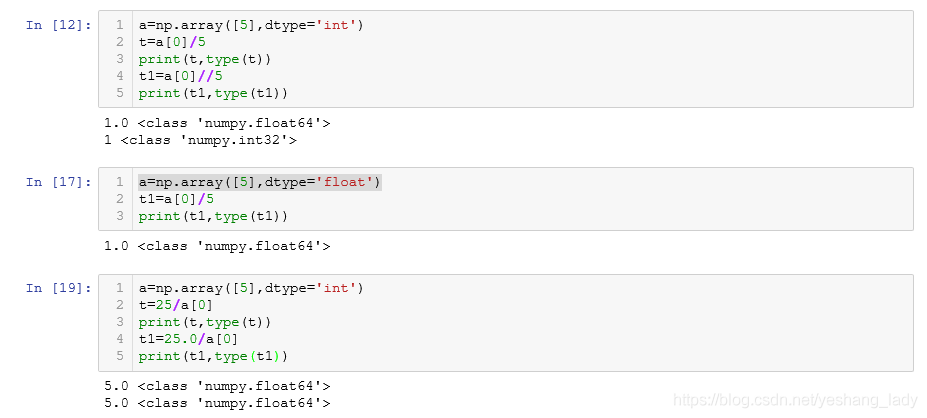# A = np.column_stack((x_vals_column, ones_column))

b = np.row_stack((x_vals_column, ones_column))

# flatten()函数用法

flatten是numpy.ndarray.flatten的一个函数，即返回一个一维数组。
flatten只能适用于numpy对象，即array或者mat，普通的list列表不适用！。
a.flatten()：a是个数组，a.flatten()就是把a降到一维，默认是按行的方向降 。
a.flatten().A：a是个矩阵，降维后还是个矩阵，矩阵.A（等效于矩阵.getA()）变成了数组。

``````>>> a=mat([[1,2,3],[4,5,6]])
>>> a
matrix([[1, 2, 3],
[4, 5, 6]])
>>> a.flatten()
matrix([[1, 2, 3, 4, 5, 6]])
>>> y=a.flatten().A
>>> shape(y)
(1L, 6L)
>>> shape(y)
(6L,)
>>> a.flatten().A
array([1, 2, 3, 4, 5, 6])
>>>
``````

# set() 函数

set() 函数创建一个无序不重复元素集，可进行关系测试，删除重复数据，还可以计算交集、差集、并集等。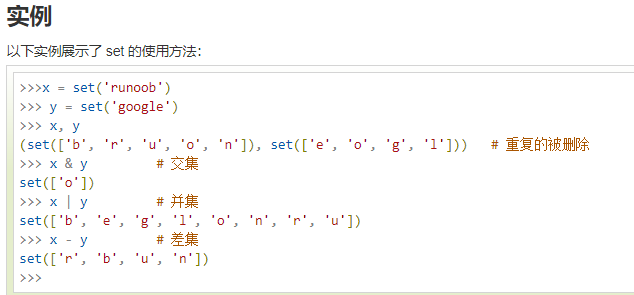# np.arange函数的使用

1.只有一个参数的时候，默认从0开始到输入的参数，产生序列，步进为默认的1
2.有两个参数的时候，序列中的元素从第一个参数到第二个参数的区间产生，步进为默认的1
3.有三个参数的时候，序列中的元素从第一个参数到第二个参数的区间产生，步进为第三个参数

# numpy.astype() 转换数据类型

arr.astype(int32) 将arr（array数组）转化为int32类型
int32 --> float64 完全ojbk
float64 --> int32 会将小数部分截断
string_ --> float64 如果字符串数组表示的全是数字，也可以用astype转化为数值类型

# np.tile

b = tile(a,(m,n)):即是把a数组里面的元素复制n次放进一个数组c中，然后再把数组c复制m次放进一个数组b中

# sum(axis=1)

axis=1表示按行相加 , axis=0表示按列相加

# Numpy tolist() 用法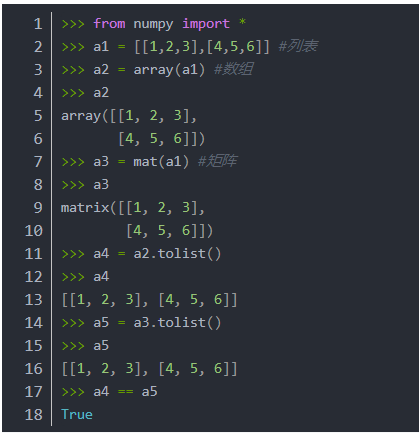# np.c_的用法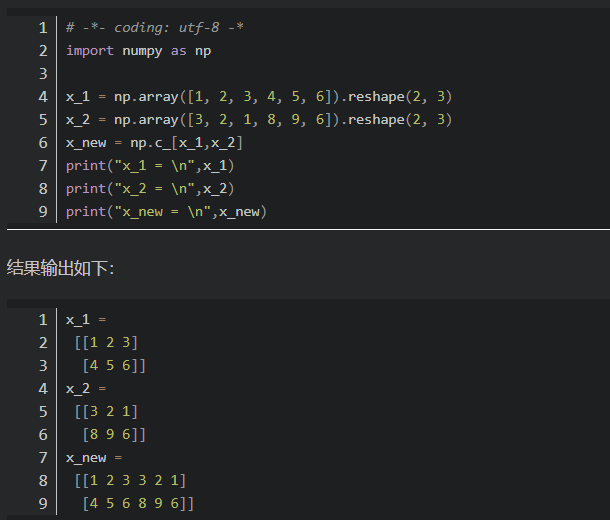# np.argmax

numpy.argmax(array, axis) 用于返回一个numpy数组中最大值的索引值。当一组中同时出现几个最大值时，返回第一个最大值的索引值。

# 查准率(Precision) 、召回率(Recall)与F1分数、准确率(Accuracy) | 查准率(Precision) | 查全率(Recall)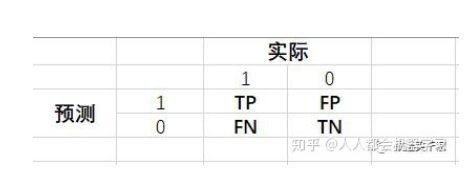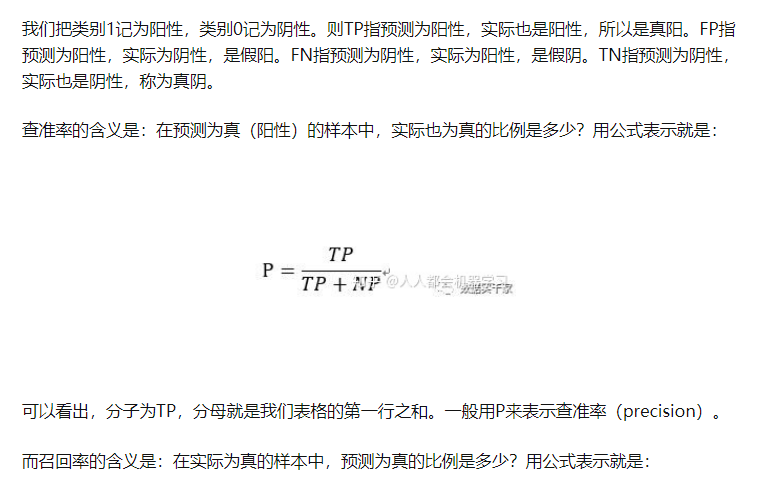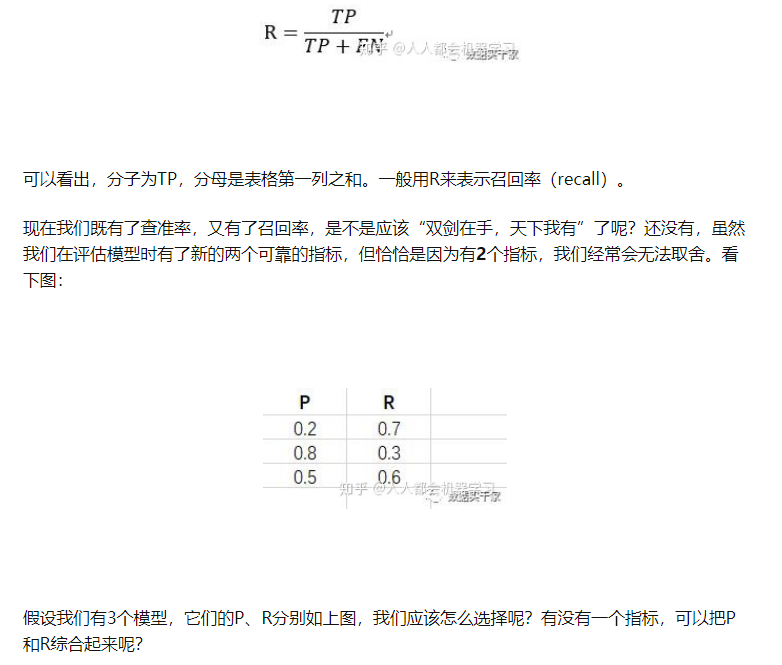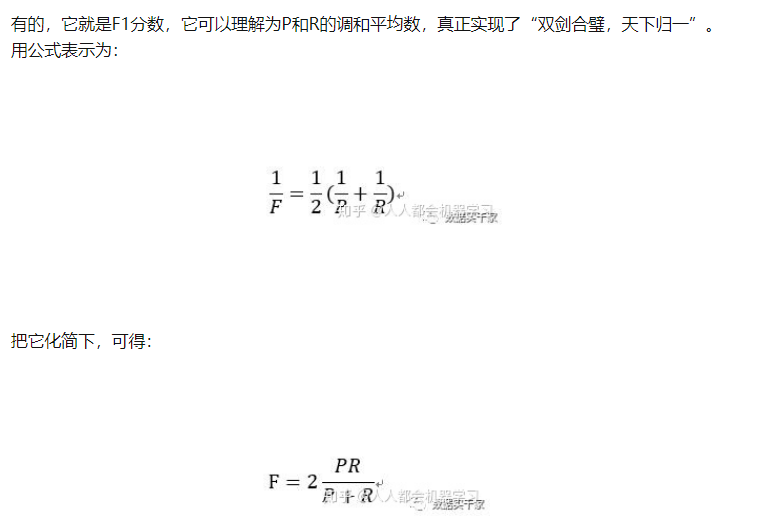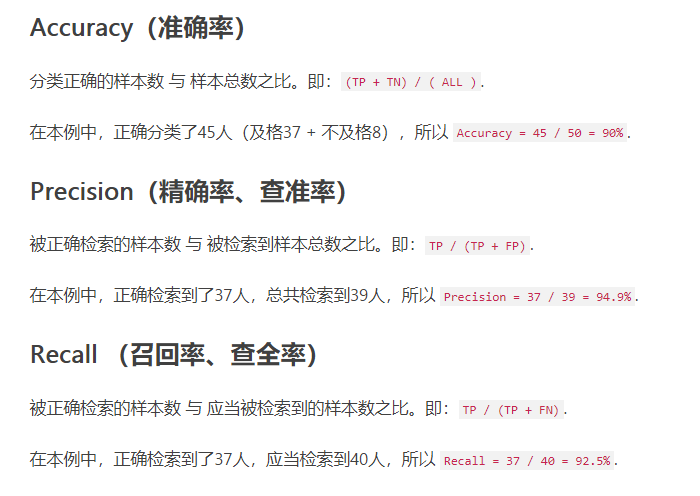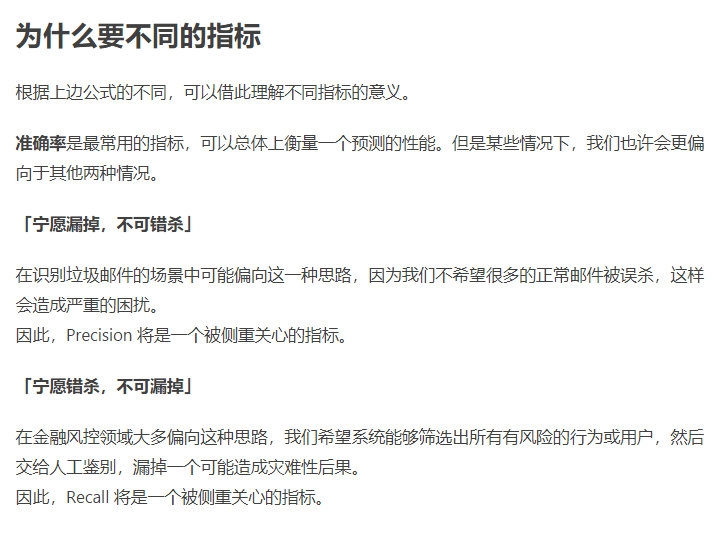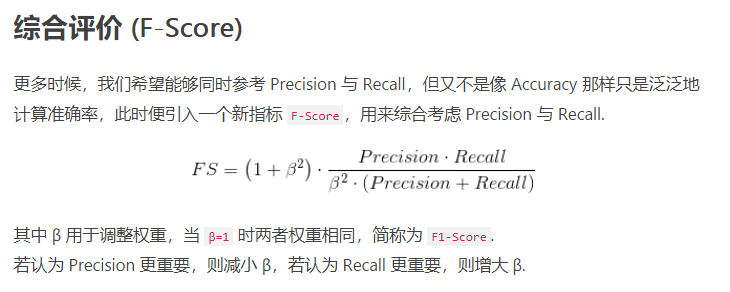# Recall（召回率） Precision（准确率） F-Measure E值 sensitivity（灵敏性） specificity（特异性）漏诊率 误诊率 ROC AUC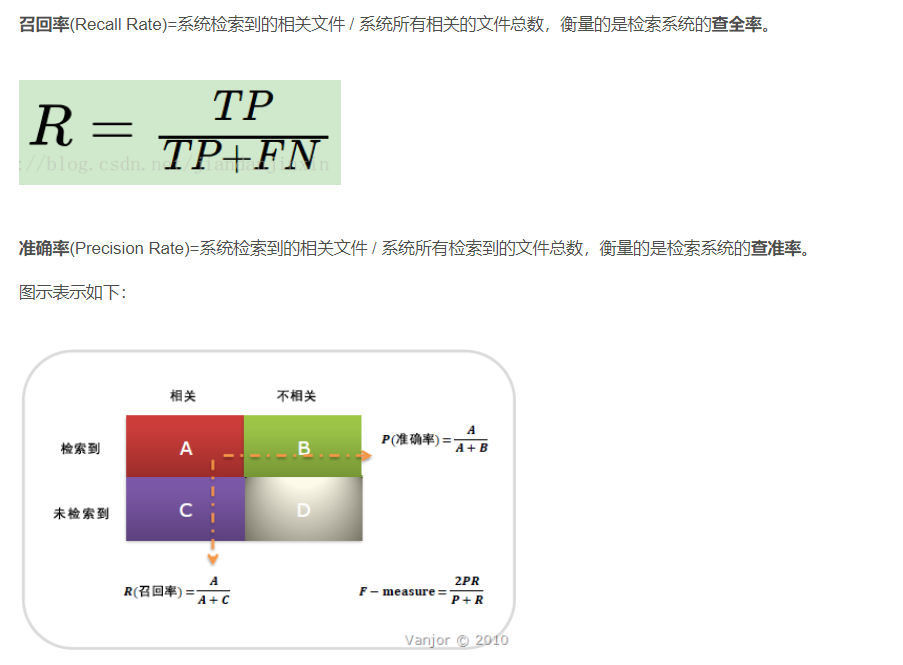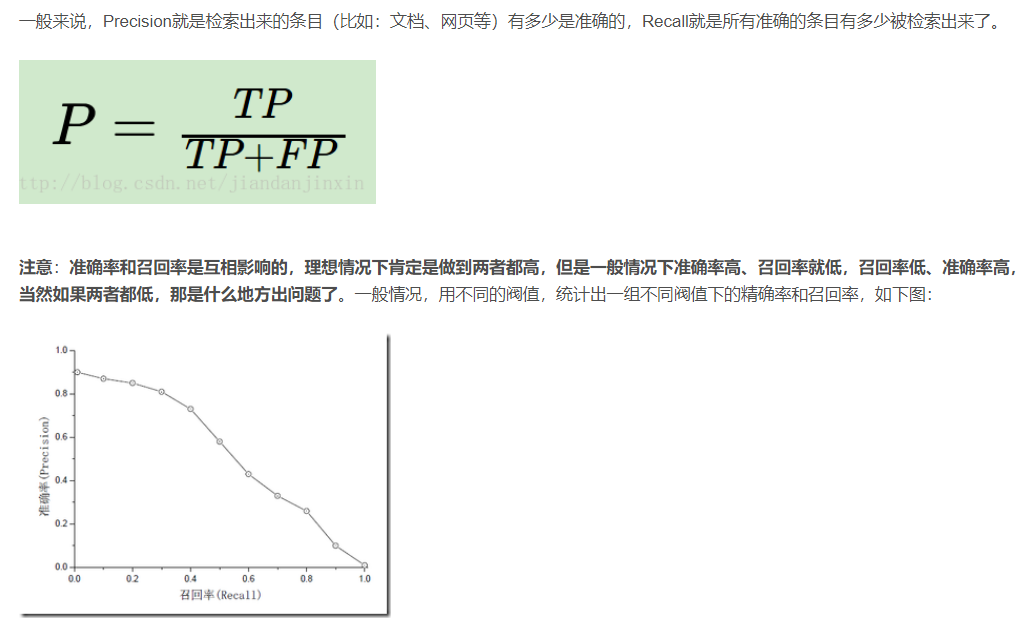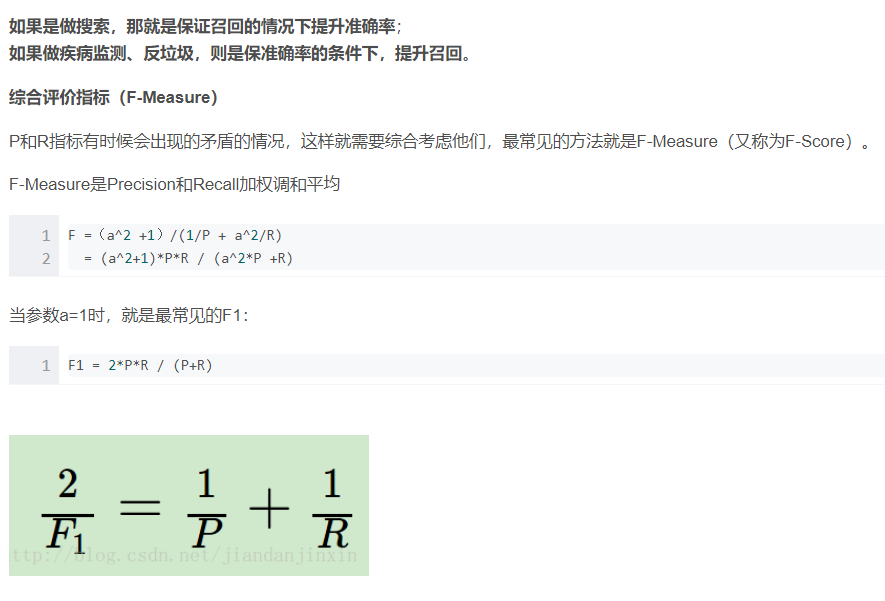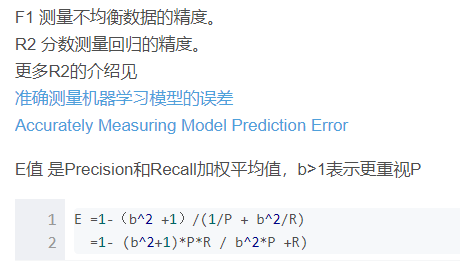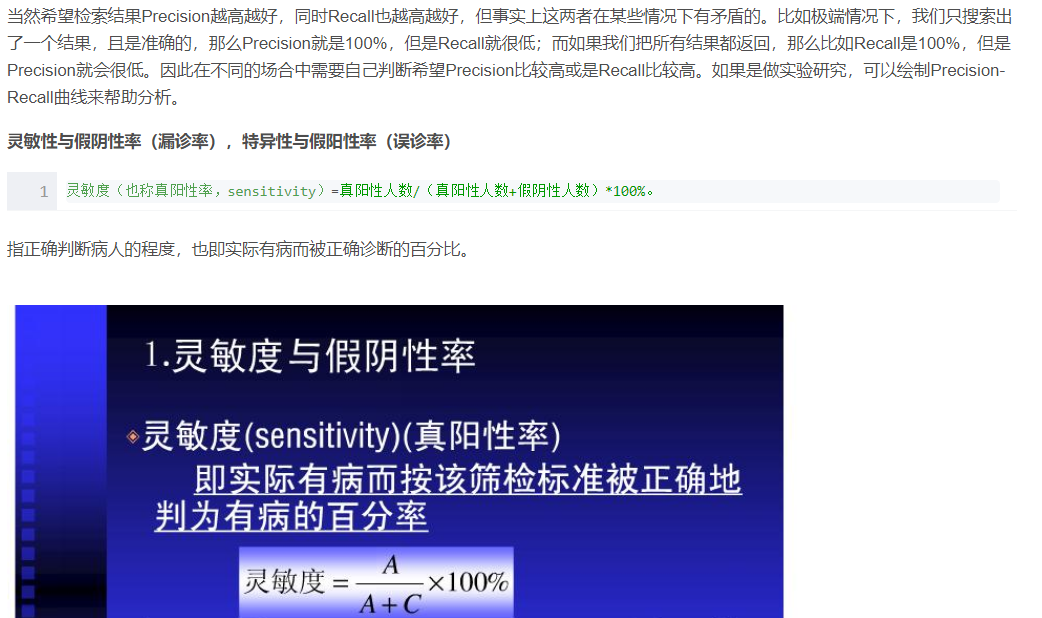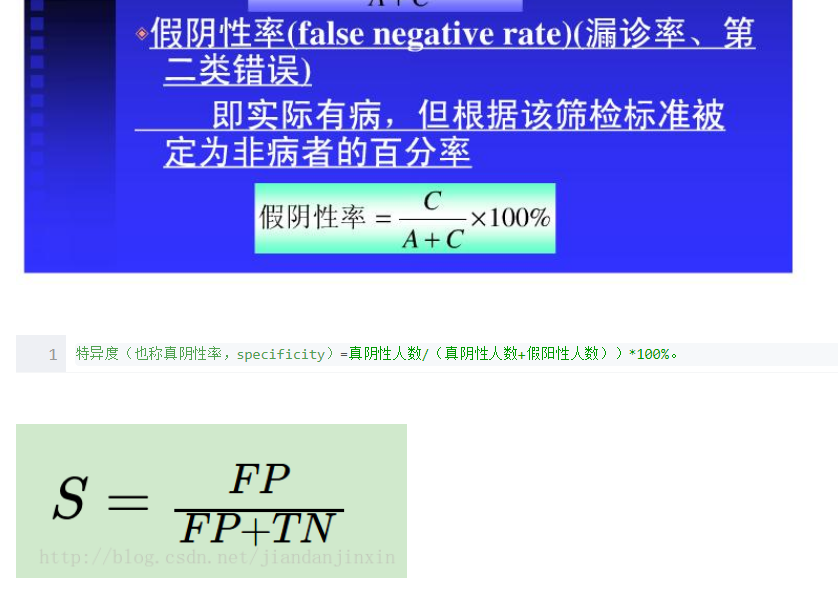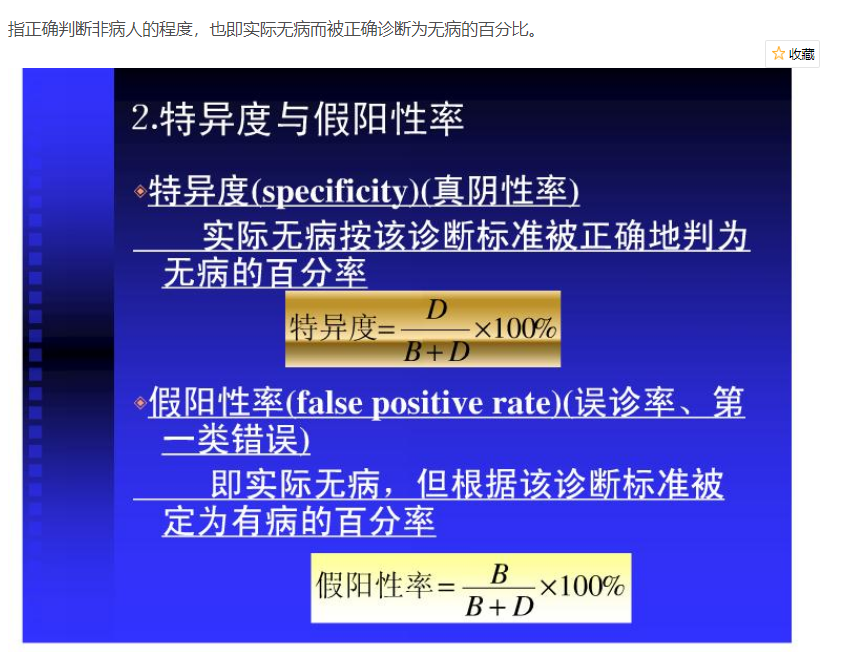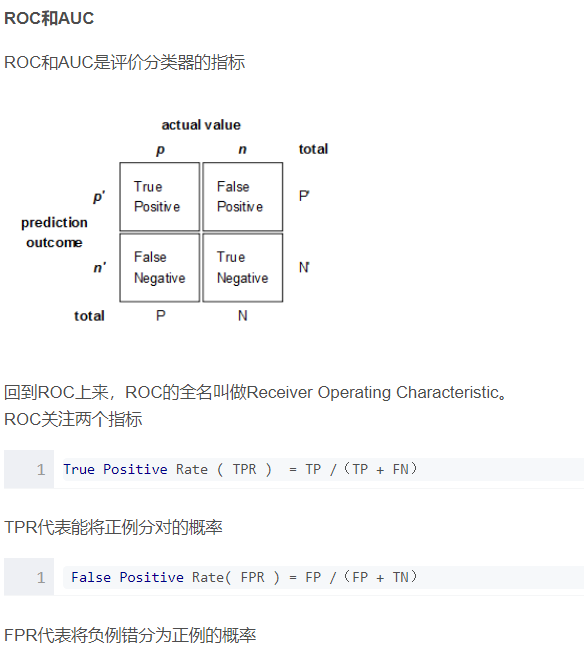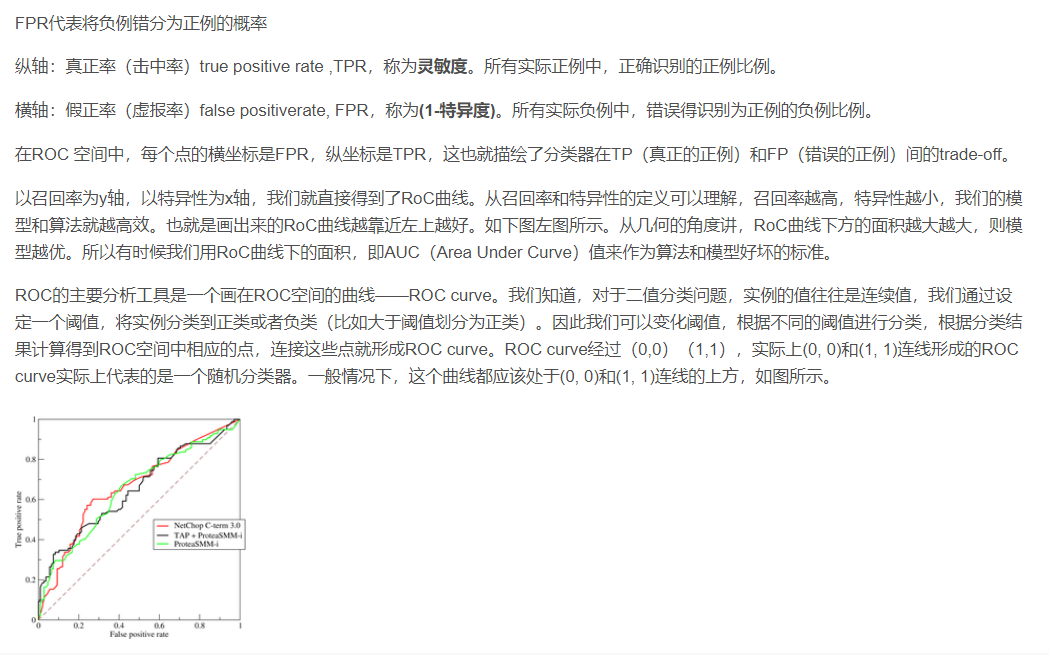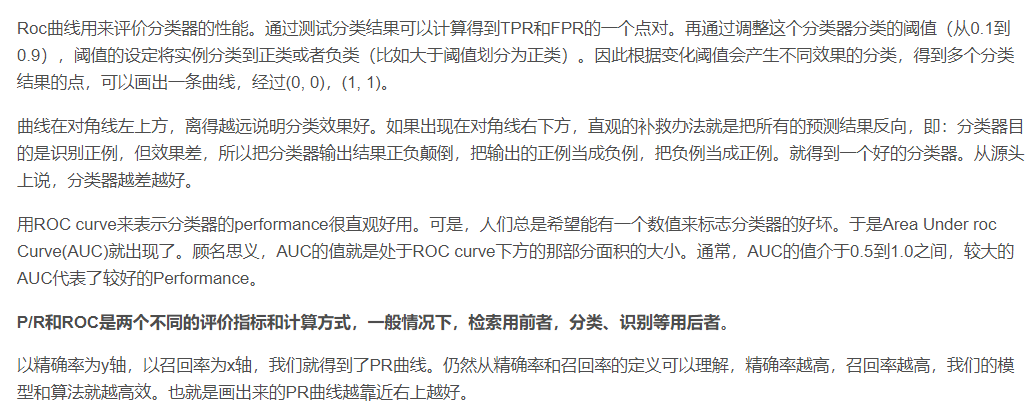# numpy.around()函数

a为输入列表或矩阵；
decimals为n对输入近似后保留小数点后n位，默认为0，若值为-n，则对小数点左边第n位近似；
out为可选参数，一般不用，用于保存近似返回结果。

# python csv.reader() 与 pd.read_csv()的区别

## csv.reader()

``````import pandas as pd

r = []
with open('train.csv',encoding = 'utf-8') as text:
row = csv.reader(text, delimiter = ',')
for r in row:
print(r)

['姓名', '数学', '语文', '英语']
['小王', '54', '76', '87']
['小李', '32', '34', '69']
['小刚', '78', '28', '77']
#输出的是一行行列表
``````

## pd.read_csv()

``````data = pd.read_csv('train.csv',encoding='utf-8')
print(data)

姓名  数学  语文  英语
0  小王  54  76  87
1  小李  32  34  69
2  小刚  78  28  77
``````

# sp.linalg.solve_sylvester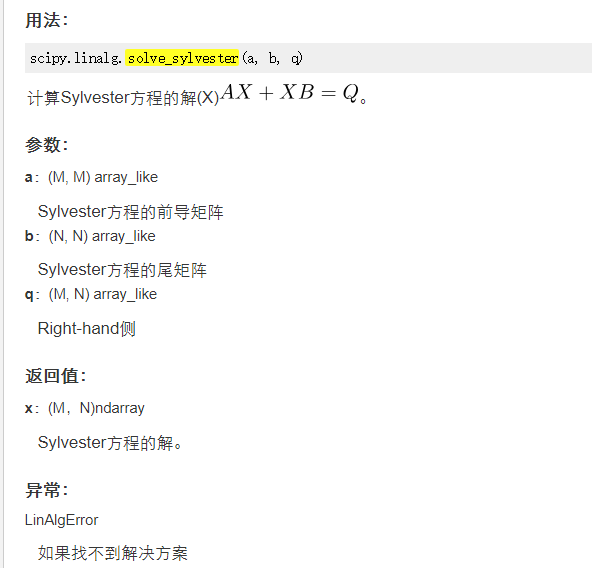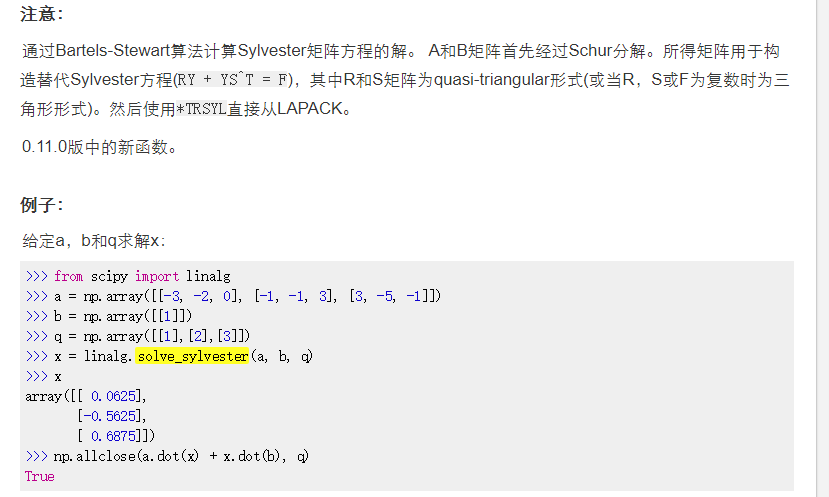# numpy.diagflat(a，k = 0)：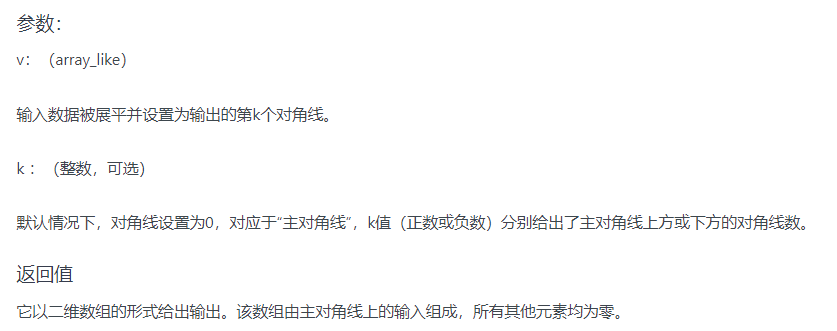# iloc和loc

loc函数：通过行索引 “Index” 中的具体值来取行数据（如取"Index"为"A"的行）
iloc函数：通过行号来取行数据（如取第二行的数据）

# pickle.dump

pickle.dumps()将对象obj对象序列化并返回一个byte对象
pickle.dump(obj, file)
pickle.dump() 直接把对象序列化后，将对象obj保存到文件file(这里的file是文件句柄) 中去。

``````import pickle
dict1 = dict(name='八岐大蛇',
age=1000,
sex='男',
addr='东方',
enemy=['八神', '草薙京', '神乐千鹤'])
# print(dict1)
data_dumps = pickle.dumps(dict1)
print(data_dumps)#b'\x80\x03}q\x00(X\x04\x00\x00\x00nameq\x01X\x0c\x00\x00\x00\xe5\x85\xab\xe5\xb2\x90\xe5\xa4\xa7\xe8\x9b\x87q\x02X\x03\x00\x00\x00ageq\x03M\xe8\x03X\x03\x00\x00\x00sexq\x04X\x03\x00\x00\x00\xe7\x94\xb7q\x05X\x04\x00\x00\x00addrq\x06X\x06\x00\x00\x00\xe4\xb8\x9c\xe6\x96\xb9q\x07X\x05\x00\x00\x00enemyq\x08]q\t(X\x06\x00\x00\x00\xe5\x85\xab\xe7\xa5\x9eq\nX\t\x00\x00\x00\xe8\x8d\x89\xe8\x96\x99\xe4\xba\xacq\x0bX\x0c\x00\x00\x00\xe7\xa5\x9e\xe4\xb9\x90\xe5\x8d\x83\xe9\xb9\xa4q\x0ceu.'

print(type(data_dumps))  # <class 'bytes'>
data=pickle.loads(data_dumps )#从字节对象中读取被封装的对象，并返回
print(data)#{'name': '八岐大蛇', 'age': 1000, 'sex': '男', 'addr': '东方', 'enemy': ['八神', '草薙京', '神乐千鹤']}
``````